# Using MONTH and EOMONTH functions in Excel - formula examples

The tutorial explains the nuts and bolts of Excel MONTH and EOMONTH functions. You will find an array of formula examples demonstrating how to extract month from date in Excel, get the first and last day of the month, convert month name to number and more.

In the previous article, we explored a variety of Excel functions to work with weekdays and days of year. Today, we are going to operate on a bigger time unit and learn the functions that Microsoft Excel provides for months.

In this tutorial, you will learn:

## Excel MONTH function - syntax and uses

Microsoft Excel provides a special MONTH function to extract a month from date, which returns the month number ranging from 1 (January) to 12 (December).

The MONTH function can be used in all versions of Excel 2016 - 2000 and its syntax is as simple as it can possibly be:

MONTH(serial_number)

Where `serial_number` is any valid date of the month you are trying to find.

For the correct work of Excel MONTH formulas, a date should be entered by using the DATE(year, month, day) function. For example, the formula `=MONTH(DATE(2015,3,1))` returns 3 since DATE represents the 1st day of March, 2015.

Formulas like `=MONTH("1-Mar-2015")` also work fine, though problems may occur in more complex scenarios if dates are entered as text.

In practice, instead of specifying a date within the MONTH function, it's more convenient to refer to a cell with a date or supply a date returned by some other function. For example:

`=MONTH(A1)` - returns the month of a date in cell A1.

`=MONTH(TODAY())` - returns the number of the current month.

At first sight, the Excel MONTH function may look plain. But look through the below examples and you will be amazed to know how many useful things it can actually do.

## How to get month number from date in Excel

There are several ways to get month from date in Excel. Which one to choose depends on exactly what result you are trying to achieve.

1. MONTH function in Excel - get month number from date.

This is the most obvious and easiest way to convert date to month in Excel. For example:

• `=MONTH(A2)` - returns the month of a date in cell A2.
• `=MONTH(DATE(2015,4,15))` - returns 4 corresponding to April.
• `=MONTH("15-Apr-2015")` - obviously, returns number 4 too.
2. TEXT function in Excel - extract month as a text string.

An alternative way to get a month number from an Excel date is using the TEXT function:

• `=TEXT(A2, "m")` - returns a month number without a leading zero, as 1 - 12.
• `=TEXT(A2,"mm")` - returns a month number with a leading zero, as 01 - 12.

Please be very careful when using TEXT formulas, because they always return month numbers as text strings. So, if you plan to perform some further calculations or use the returned numbers in other formulas, you'd better stick with the Excel MONTH function.

The following screenshot demonstrates the results returned by all of the above formulas. Please notice the right alignment of numbers returned by the MONTH function (cells C2 and C3) as opposed to left-aligned text values returned by the TEXT functions (cells C4 and C5).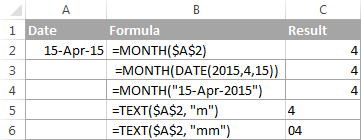## How to extract month name from date in Excel

In case you want to get a month name rather than a number, you use the TEXT function again, but with a different date code:

• `=TEXT(A2, "mmm")` - returns an abbreviated month name, as Jan - Dec.
• `=TEXT(A2,"mmmm")` - returns a full month name, as January - December.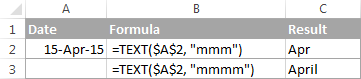If you don't actually want to convert date to month in your Excel worksheet, you are just wish to display a month name only instead of the full date, then you don't want any formulas.

Select a cell(s) with dates, press Ctrl+1 to opent the Format Cells dialog. On the Number tab, select Custom and type either "mmm" or "mmmm" in the Type box to display abbreviated or full month names, respectively. In this case, your entries will remain fully functional Excel dates that you can use in calculations and other formulas. For more details about changing the date format, please see Creating a custom date format in Excel.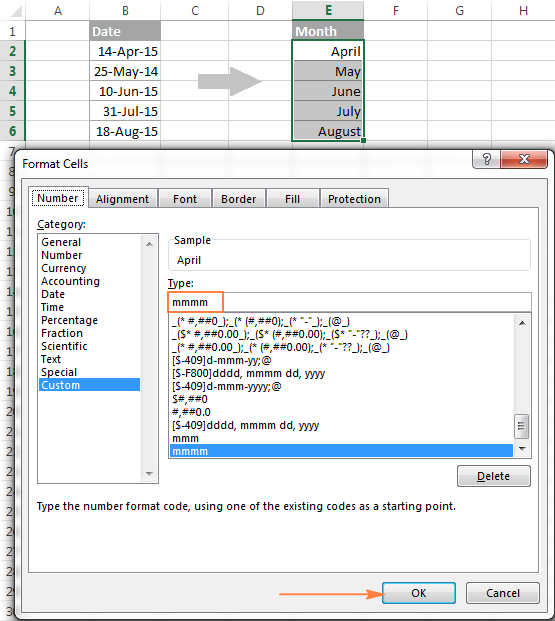## How to convert month number to month name in Excel

Suppose, you have a list of numbers (1 through 12) in your Excel worksheet that you want to convert to month names. To do this, you can use any of the following formulas:

1. To return an abbreviated month name (Jan - Dec).

`=TEXT(A2*28, "mmm")`

`=TEXT(DATE(2015, A2, 1), "mmm")`

2. To return a full month name (January - December).

`=TEXT(A2*28, "mmmm")`

`=TEXT(DATE(2015, A2, 1), "mmmm")`

In all of the above formulas, A2 is a cell with a month number. And the only real difference between the formulas is the month codes:

• "mmm" - 3-letter abbreviation of the month, such as Jan - Dec
• "mmmm" - month spelled out completely
• "mmmmm" - the first letter of the month name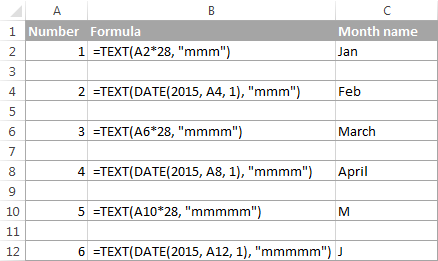### How these formulas work

When used together with month format codes such as "mmm" and "mmmm", Excel considers the number 1 as Day 1 in January 1900. Multiplying 1, 2, 3 etc. by 28, you are getting Days 28, 56, 84, etc. of the year 1900, which are in January, February, March, etc. The format code "mmm" or "mmmm" displays only the month name.

## How to convert month name to number in Excel

There are two Excel functions that can help you convert month names to numbers - DATEVALUE and MONTH. Excel's DATEVALUE function converts a date stored as text to a serial number that Microsoft Excel recognizes as a date. And then, the MONTH function extracts a month number from that date.

The complete formula is as follows:

`=MONTH(DATEVALUE(A2 & "1"))`

Where A2 in a cell containing the month name you want to turn into a number (&"1" is added for the DATEVALUE function to understand it's a date).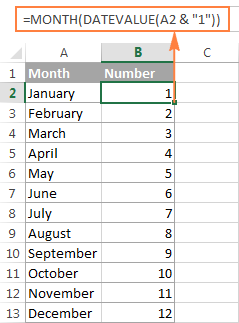## How to get the last day of month in Excel (EOMONTH function)

The EOMONTH function in Excel is used to return the last day of the month based on the specified start date. It has the following arguments, both of which are required:

EOMONTH(start_date, months)
• Start_date - the starting date or a reference to a cell with the start date.
• Months - the number of months before or after the start date. Use a positive value for future dates and negative value for past dates.

Here are a few EOMONTH formula examples:

`=EOMONTH(A2, 1)` - returns the last day of the month, one month after the date in cell A2.

`=EOMONTH(A2, -1)` - returns the last day of the month, one month before the date in cell A2.

Instead of a cell reference, you can hardcode a date in your EOMONTH formula. For example, both of the below formulas return the last day in April.

`=EOMONTH("15-Apr-2015", 0)`

`=EOMONTH(DATE(2015,4,15), 0)`

To return the last day of the current month, you use the TODAY() function in the first argument of your EOMONTH formula so that today's date is taken as the start date. And, you put 0 in the `months` argument because you don't want to change the month either way.

`=EOMONTH(TODAY(), 0)`

Note. Since the Excel EOMONTH function returns the serial number representing the date, you have to apply the date format to a cell(s) with your formulas. Please see How to change date format in Excel for the detailed steps.

And here are the results returned by the Excel EOMONTH formulas discussed above: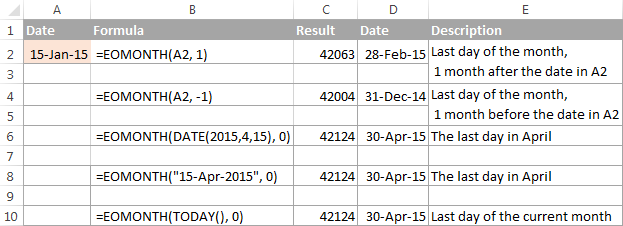If you want to calculate how many days are left till the end of the current month, you simply subtract the date returned by TODAY() from the date returned by EOMONTH and apply the General format to a cell:

`=EOMONTH(TODAY(), 0)-TODAY()`

## How to find the first day of month in Excel

As you already know, Microsoft Excel provides just one function to return the last day of the month (EOMONTH). When it comes to the first day of the month, there is more than one way to get it.

#### Example 1. Get the 1st day of month by the month number

If you have the month number, then use a simple DATE formula like this:

=DATE(year, month number, 1)

For example, =DATE(2015, 4, 1) will return 1-Apr-15.

If your numbers are located in a certain column, say in column A, you can add a cell reference directly in the formula:

`=DATE(2015, B2, 1)`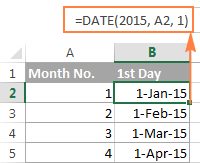#### Example 2. Get the 1st day of month from a date

If you want to calculate the first day of the month based on a date, you can use the Excel DATE function again, but this time you will also need the MONTH function to extract the month number:

=DATE(year, MONTH(cell with the date), 1)

For example, the following formula will return the first day of the month based on the date in cell A2:

`=DATE(2015,MONTH(A2),1)`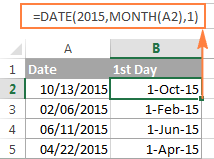#### Example 3. Find the first day of month based on the current date

When your calculations are based on today's date, use a liaison of the Excel EOMONTH and TODAY functions:

`=EOMONTH(TODAY(),0) +1` - returns the 1st day of the following month.

As you remember, we already used a similar EOMONTH formula to get the last day of the current month. And now, you simply add 1 to that formula to get the first day of the next month.

In a similar manner, you can get the first day of the previous and current month:

`=EOMONTH(TODAY(),-2) +1` - returns the 1st day of the previous month.

`=EOMONTH(TODAY(),-1) +1` - returns the 1st day of the current month.

You could also use the Excel DATE function to handle this task, though the formulas would be a bit longer. For example, guess what the following formula does?

`=DATE(YEAR(TODAY()), MONTH(TODAY()), 1)`

Yep, it returns the first day of the current month.

And how do you force it to return the first day of the following or previous month? Hands down :) Just add or subtract 1 to/from the current month:

To return the first day of the following month:

`=DATE(YEAR(TODAY()), MONTH(TODAY())+1, 1)`

To return the first day of the previous month:

`=DATE(YEAR(TODAY()), MONTH(TODAY())-1, 1)`

## How to calculate the number of days in a month

In Microsoft Excel, there exist a variety of functions to work with dates and times. However, it lacks a function for calculating the number of days in a given month. So, we'll need to make up for that omission with our own formulas.

#### Example 1. To get the number of days based on the month number

If you know the month number, the following DAY / DATE formula will return the number of days in that month:

=DAY(DATE(year, month number + 1, 1) -1)

In the above formula, the DATE function returns the first day of the following month, from which you subtract 1 to get the last day of the month you want. And then, the DAY function converts the date to a day number.

For example, the following formula returns the number of days in April (the 4th month in the year).

`=DAY(DATE(2015, 4 +1, 1) -1)`

#### Example 2. To get the number of days in a month based on date

If you don't know a month number but have any date within that month, you can use the YEAR and MONTH functions to extract the year and month number from the date. Just embed them in the DAY / DATE formula discussed in the above example, and it will tell you how many days a given month contains:

`=DAY(DATE(YEAR(A2), MONTH(A2) +1, 1) -1)`

Where A2 is cell with a date.

Alternatively, you can use a much simpler DAY / EOMONTH formula. As you remember, the Excel EOMONTH function returns the last day of the month, so you don't need any additional calculations:

`=DAY(EOMONTH(A1, 0))`

The following screenshot demonstrates the results returned by all of the formulas, and as you see they are identical: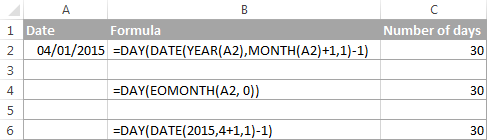## How to sum data by month in Excel

In a large table with lots of data, you may often need to get a sum of values for a given month. And this might be a problem if the data was not entered in chronological order.

The easiest solution is to add a helper column with a simple Excel MONTH formula that will convert dates to month numbers. Say, if your dates are in column A, you use =MONTH(A2).

And now, write down a list of numbers (from 1 to 12, or only those month numbers that are of interest to you) in an empty column, and sum values for each month using a SUMIF formula similar to this:

`=SUMIF(C2:C15, E2, B2:B15)`

Where E2 is the month number.

The following screenshot shows the result of the calculations: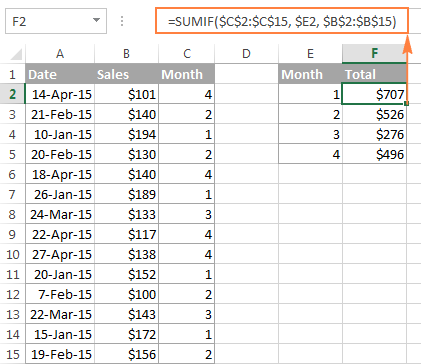If you'd rather not add a helper column to your Excel sheet, no problem, you can do without it. A bit more trickier SUMPRODUCT function will work a treat:

`=SUMPRODUCT((MONTH(\$A\$2:\$A\$15)=\$E2) * (\$B\$2:\$B\$15))`

Where column A contains dates, column B contains the values to sum and E2 is the month number.

Note. Please keep in mind that both of the above solutions add up all values for a given month regardless of the year. So, if your Excel worksheet contains data for several years, all of it will be summed.

## How to conditionally format dates based on month

Now that you know how to use the Excel MONTH and EOMONTH functions to perform various calculations in your worksheets, you may take a step further and improve the visual presentation. For this, we are going to use the capabilities of Excel conditional formatting for dates.

In addition to the examples provided in the above mentioned article, now I will show you how you can quickly highlight all cells or entire rows related to a certain month.

#### Example 1. Highlight dates within the current month

In the table from the previous example, suppose you want to highlight all rows with the current month dates.

First off, you extract the month numbers from dates in column A using the simplest =MONTH(\$A2) formula. And then, you compare those numbers with the current month returned by =MONTH(TODAY()). As a result, you have the following formula which returns TRUE if the months' numbers match, FALSE otherwise:

`=MONTH(\$A2)=MONTH(TODAY())`

Create an Excel conditional formatting rule based on this formula, and your result may resemble the screenshot below (the article was written in April, so all April dates are highlighted).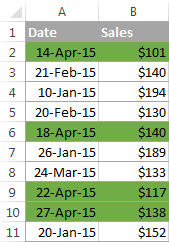#### Example 2. Highlighting dates by month and day

And here's another challenge. Suppose you want to highlight the major holidays in your worksheet regardless of the year. Let's say Christmas and New Year days. How would you approach this task?

Simply use the Excel DAY function to extract the day of the month (1 - 31) and the MONTH function to get the month number, and then check if the DAY is equal to either 25 or 31, and if the MONTH is equal to 12:

`=AND(OR(DAY(\$A2)=25, DAY(\$A2)=31), MONTH(A2)=12)`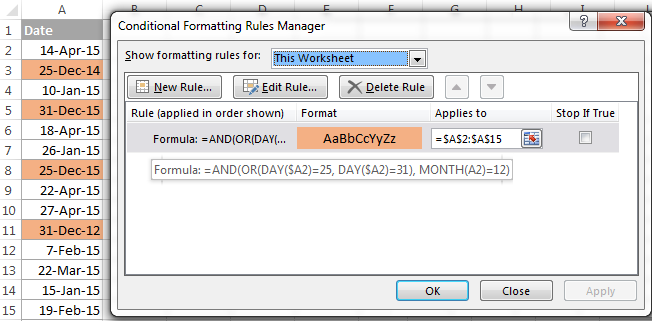This is how the MONTH function in Excel works. It appears to be far more versatile than it looks, huh?

In a couple of the next posts, we are going to calculate weeks and years and hopefully you will learn a few more useful tricks. If you are interested in smaller time units, please check out the previous parts of our Excel Dates series (you will find the links below). I thank you for reading and hope to see you next week!

-->

### 152 Responses to "Using MONTH and EOMONTH functions in Excel - formula examples"

1.virender says:

how calculate the sum 31 as a month or how to show 31 as (1 month or 1) in excel
i try =DATEDIF(0,F25,"ym") (where F25 is a no. or sum =31) but is show only 31 as result instead of 1.

2.virender says:

if i have a sum or number like 28, 30 or 31 how i count these no. as 1 month
i try formula =DATEDIF(0,F26,"ym") but it show 0 and formula =DATEDIF(0,F26,"md") it show same no. as 28, 30 & 31.
i want it show as 1 month if any solution or formula pls.

3.KP says:

I have dates in a row (dates span across months) and certain values in the cells below these dates (e.g. work shifts such as "M" for morning). I need to count number of occurrences of a shift per month. How to I achieve this ?
I tried using MONTH() and COUNTIF()/COUNTIFS() together but failed.

4.Alok Ghosh says:

I have different dates in column A1 (1-Oct-19, 15-Oct-19,29-Oct-19, 12-Nov-19, 26-Nov-19, 10-Jan-21 and so on). I want to highlight only the current month in green and the rest of the month in the red. I mean before and after Jan in the red and Jan (Current Month) in green.

•Alok Ghosh says:

Example 1. Highlight dates within the current month
=MONTH(\$A2)=MONTH(TODAY())
Through this, if year changes it is highlighting the same month also. How can I highlight the date within the current month and year?
10-Jan-20, 15-Jan-21
It will highlight both cells. When the year is 2020, it should not highlight the year 2021.

5.Alok Ghosh says:

Problem solved:
I used this-
=AND(MONTH(\$A1)=MONTH(TODAY()),YEAR(\$A1)=YEAR(TODAY()))

•Alok Ghosh says:

Problem solved:
I used this-
=AND(MONTH(\$A1)=MONTH(TODAY()),YEAR(\$A1)=YEAR(TODAY()))
Now I want the rest of the date should be highlighted in yellow colour. I mean if the month and the year is less than or more than today's month and the year it should be highlighted in yellow colour.

6.thou says:

hi
i have one question, i have the calendar in excel and i am entering holidays next to the particular date. when i change the month, how i can clear the my holiday entries automatically.
thanks

7.Michael says:

I am facing a problem that I am using to display a stacked bar chart with "Time" as the timeline. However, I don't know how to make the x-axis (timeline) to display 1st day of each month. Any idea?

8.Sam says:

Hi,
Struggled to understand this, =TEXT(A2*28, "mmmm"). Why multiply by 28?

•Svetlana Cheusheva (Ablebits.com Team) says:

Hi Sam,

Thank you for a very good question!

In its internal system, Excel considers the number 1 as Day 1 in January 1900 (when used together with month format codes such as "mmm" and "mmmm"). By multiplying the numbers 1, 2, 3 etc. by 28, you are getting Days 28, 56, 84, etc. which are in January, February, March, etc. Multiplying by 29 will also work.

9.Suresh Laljibhai Vaghela says:

I have few date range with different months and year. I want to hight only date which are from January to June regardless year. Can you please help me for this?

Example.
04-12-2006
05-06-2007
20-08-2008
05-01-2009
09-11-2007
25-06-2008
08-01-2009
24-06-2008
01-02-2011
17-11-2011
24-09-2011
05-09-2012
30-09-2011
24-09-2011
04-07-2019
04-01-2009
31-12-2013
01-12-2013
01-12-2013
01-12-2013
22-01-2014
25-06-2008
01-12-2013
17-02-2012
01-12-2013
12-03-2016
12-03-2016
12-03-2016
12-03-2016
12-03-2016
12-12-2017
07-05-2019
10-07-2019
10-06-2019
05-07-2019
05-07-2019
29-07-2019
28-09-2019
from above dates I want to hightligh only dates from January to June regardless year.

10.jaweed says:

01/01/2020 AMUH
02/01 DLD
03/01 AMUH
04/01 ARASH
05/01 DAFZA
02/02/2020 AMUH
04/02/2020 UTPM
01/03/2020 UPTM
07/03/2020 AMUH
04/04/2020 AMUH

HOW CAN WE CALCULATE MONT WISE

KINDLY SHOW THE CALCULATION

11.Mark says:

Thanks a lot for your information. I have an issue to convert the following text date to a real date type.
Jan 02, 2018
Would you help on how I can convert it to date type? I've tried "=date(RIGHT(A2,4) LEFT(A2,3) MID(A2,5,2))", but it doesn't work.

•Alexander Trifuntov (Ablebits.com Team) says:

Hello? Mark!

=DATEVALUE(A2)

12.brenda says:

I need a formal that if a month has 31 days it will displace from cell D5 and if then displace from cell D4. the month is in A1. Can you help me, please?

•Alexander Trifuntov (Ablebits.com Team) says:

Hello, Brenda!
If I understand your task correctly, the following formula should work for you:

=IF(DAY (EOMONTH (DATE(2020,A1,1),0)) = 31, D5, D4)

13.Michael W Smith Jr says:

Hello,
I have calculated the number of year and months in excel. Now I am trying to get Excel to tell me what month and year that will be from now. For example, my car note will be paid off 2 years and 9 months from now, March 29, 2020. How can I get excel to tell me that 2 years and 9 months from now will be in December 2022? I thank you for your help with respect to resolving this issue in advance.

14.Alexander Trifuntov (Ablebits.com Team) says:

Hello Michael!
=DATE(YEAR(A1)+2, MONTH(A1)+9, DAY(A1)+0) or
=DATE(YEAR(TODAY())+2, MONTH(TODAY())+9, DAY(TODAY())+0)
This tool is available as a part of our Ultimate Suite for Excel that you can install in a trial mode and use for 30 days for free: https://www.ablebits.com/files/get.php?addin=xl-suite&f=free-trial

15.Carl says:

I need to take a date from this format 3/31/2020 to the "31st day of March 2020". I have NO problem on the March 2020 part but HOW in the world can I get the st part of 31st ??? Also need the abbreviations for all days 1, 2, 3, ..... 29, 30, and 31. Like 1st. 2nd, 3rd, and so on. The Only workaround I can think of is to create a table or chart with all the possible numbers with abbreviations and then use vlookup or the new xlookup.

Thanks in advance for any help or insight !!!

•Alexander Trifuntov (Ablebits.com Team) says:

Hello Carl!
If I understand your task correctly, the following formula convert to ordinal date format:

=DAY(A1)&IF(OR(DAY(A1) = {1,2,3,21,22,23,31}),CHOOSE (1*RIGHT(DAY(A1),1),"st ","nd ","rd "),"th")&TEXT(A1,"mmmm, yyyy")
or
=DAY(A1)&IF(OR(DAY(A1) = {1,2,3,21,22,23,31}),CHOOSE (1*RIGHT(DAY(A1),1),"st day of ","nd day of ","rd day of "),"th day of ")&TEXT(A1,"mmmm, yyyy")

16.Himanshu says:

I have downloaded a data which is from Jan-2020 to mar 2020, like below:
05/02/2020 06:09 - It is showing month as May
1/31/2020 2:55 AM - This one is showing Jan
1/29/2020 12:27 AM
1/17/2020 6:47 AM
1/16/2020 5:39 AM
1/14/2020 7:46 AM
06/01/2020 03:41 - This one showing as June 20202.
I tried converting it into month through text formula, tried changing format through data tab to convert text to column, also manually tried custom formatting but no go.
•Alexander Trifuntov (Ablebits.com Team) says: